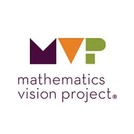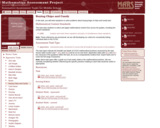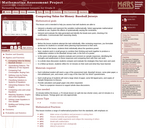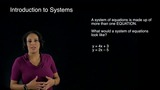Updating search results...

# 27 Results

View
Selected filters:
• NC.M1.A-CED.3Conditional Remix & Share Permitted
CC BY-NC-SA
Rating
0.0 stars

Students recognize and identify solutions to two-variable equations. They represent the solution set graphically. They create two variable equations to represent a situation. They understand that the graph of the line ax + by = c is a visual representation of the solution set to the equation ax + by = c.

Subject:
Math 1
Mathematics
Material Type:
Lesson
Author:
EngageNY
02/02/2020Conditional Remix & Share Permitted
CC BY-NC-SA
Rating
0.0 stars

Students use systems of equations or inequalities to solve contextual problems and interpret solutions within a particular context.

Subject:
Math 1
Mathematics
Material Type:
Lesson
Author:
EngageNY
02/02/2020Conditional Remix & Share Permitted
CC BY-NC-SA
Rating
0.0 stars

Students learn the meaning and notation of recursive sequences in a modeling setting.
Students use recursive sequences to model and answer problems.
Students create equations and inequalities to solve a modeling problem.
Students represent constraints by equations and inequalities and interpret solutions as viable or non-viable options in a modeling context.

Subject:
Math 1
Mathematics
Material Type:
Lesson
Author:
EngageNY
02/03/2020Conditional Remix & Share Permitted
CC BY-NC-SA
Rating
0.0 stars

Students create equations and inequalities in one variable and use them to solve problems.
Students create equations in two or more variables to represent relationships between quantities and graph equations on coordinate axes with labels and scales.
Students represent constraints by inequalities and interpret solutions as viable or non-viable options in a modeling context.

Subject:
Math 1
Mathematics
Material Type:
Lesson
Author:
EngageNY
02/03/2020Conditional Remix & Share Permitted
CC BY-NC-SA
Rating
0.0 stars

In the context of examining combinations of cats and dogs that satisfy all of the “Pet Sitter” constraints students will solidify the following mathematics:
• The solution set to a system of inequalities represents the set of points that satisfy all of the inequalities simultaneously. That is, a point in the solution set of a system of inequalities makes all of the inequalities true. Contexts, such as “Pet Sitters” may imply additional constraints, such as y ≥ 0 or x ≥ 0, that are not explicitly stated.
• A shaded region on the coordinate grid is used to represent the solution set for a system of inequalities in two-variables. In this case, the points on the boundary lines that trace out the region are also included in the solution set, indicated by drawing a solid line for the boundaries. A shaded polygonal region is used to represent the solution to a system of constraints.

Subject:
Math 1
Mathematics
Material Type:
Lesson
Author:
The Mathematics Vision Project
03/10/2020Rating
0.0 stars

Students use systems of equations or inequalities to solve contextual problems and interpret solutions within a particular context.

Subject:
Mathematics
Material Type:
Lesson Plan
Provider:
EngageNY
02/05/2018Rating
0.0 stars

Susie is organizing the printing of tickets for a show. She has collected prices from several printers. Your task is to use a table and algebra to advise Susie on how to choose the best printer.

Subject:
Math 1
Math 2
Math 3
Mathematics
Material Type:
Activity/Lab
Assessment
Lesson Plan
Provider:
MARS, Mathematics Assessment Resource Service
Author:
Shell Center Team
02/26/2019Only Sharing Permitted
CC BY-NC-ND
Rating
0.0 stars

In this task, students will write equations to solve problems about buying bags of chips and candy bars.

Subject:
Mathematics
Material Type:
Activity/Lab
Assessment
Author:
Mathematics Assessment Resource Service
MARS
08/11/2019Conditional Remix & Share Permitted
CC BY-NC-SA
Rating
0.0 stars

CK-12 Foundation's Algebra FlexBook is an introduction to algebraic concepts for the high school student. Topics include: Equations & Functions, Real Numbers, Equations of Lines, Solving Systems of Equations & Quadratic Equations.

Subject:
Mathematics
Material Type:
Textbook
Provider:
CK-12 Foundation
Provider Set:
CK-12 FlexBook
12/06/2018Only Sharing Permitted
CC BY-NC-ND
Rating
0.0 stars

This lesson unit is intended to help you assess how well students are able to:

* Interpret a situation and represent the variables mathematically.
* Select appropriate mathematical methods to use.
* Explore the effects of systematically varying the constraints.
* Interpret and evaluate the data generated and identify the break-even point, checking it for confirmation.
* Communicate their reasoning clearly.

Subject:
Mathematics
Material Type:
Activity/Lab
Assessment
Author:
Mathematics Assessment Resource Service
MARS
08/11/2019Rating
0.0 stars

Four guided problems for teachers and students to work through in context.

Subject:
Mathematics
Material Type:
Activity/Lab
Provider:
Cobb County School District
07/06/2018Rating
0.0 stars

SAT prep unit on systems of equations and inequalities'

Subject:
Mathematics
Material Type:
Activity/Lab
Provider:
CollegeBoard
03/05/2018Rating
0.0 stars

Students will extend their study of functions to include function notation and the concepts of domain and range by exploring examples of functions and their graphs, focusing on the contrast between linear and exponential functions. They interpret functions given graphically, numerically, symbolically, and verbally; translate between representations; and understand the limitations of various representations.

Subject:
Math 1
Math 2
Math 3
Mathematics
Material Type:
Unit of Study
Provider:
EngageNY
Author:
EngageNY
02/26/2019Conditional Remix & Share Permitted
CC BY-NC-SA
Rating
0.0 stars

The purpose of this task is to practice solving systems of linear inequalities by identifying the overlapping (or intersecting) region of the half-planes that form the solution sets of each of the two-variable inequalities in the system.

Subject:
Math 1
Mathematics
Material Type:
Lesson Plan
Author:
The Mathematics Vision Project
03/10/2020Conditional Remix & Share Permitted
CC BY-NC-SA
Rating
0.0 stars

In this series of videos, NC Virtual educator, Melissa,&nbsp;demonstrates systems of equations and systems of inequalities in a series of lightboard videos.

Subject:
Math 1
Material Type:
Presentation
Author:
Darlene Schaefer
04/15/2020Conditional Remix & Share Permitted
CC BY-NC-SA
Rating
0.0 stars

In the context of examining combinations of cats and dogs that satisfy these additional “Pet Sitter” constraints students will practice the following mathematics: (Note that each of these ideas have surfaced and have been examined and solidified in previous tasks. This is an opportunity for students to practice these ideas in a new setting.)
• Constraints can be represented by inequalities. The solution set of an inequality consists of a set of points that lie in a half-plane bounded by the equation associated with the inequality.
• Students should already have well-practiced procedures for writing equations of lines through given points from a graphical perspective. In addition, they should practice writing the equation of the boundary line using the terms defined by the constraints. Students will determine which half-plane to shade to represent the solution to the inequality by using their specific “test points” or by identifying which points are implied by the inequality sign.
• A shaded half-plane in the coordinate grid is a representation of the solution set for an inequality in two-variables. The points on the boundary line are also included in the solution set—indicated by drawing a solid line for the boundary—unless the boundary is a strict inequality and then the boundary is represented by a dotted line.

Subject:
Math 1
Mathematics
Material Type:
Lesson
Author:
The Mathematics Vision Project
03/10/2020Conditional Remix & Share Permitted
CC BY-NC-SA
Rating
0.0 stars

As students work with the context of making recommendations for how many dogs and cats Carlos and Clarita should plan to accommodate, they will surface many ideas, strategies and representations related to solving systems of equations and inequalities. For example, they will explore the notion of constraints since in this task the number of each type of pet that can be accommodated is limited by space and money, but many different combinations of dogs and cats are possible. They may consider the notion of a system of equations since each constraint (space, start-up costs) allows for a different set of possibilities—a particular combination of dogs and cats may satisfy one constraint but not another—so both constraints must be considered simultaneously. Finally, they may surface the notion of a system of inequalities since Carlos and Clarita don’t have to use up all of the available space or money, implying that each constraint may be represented by an inequality.

Subject:
Math 1
Mathematics
Material Type:
Lesson
Author:
The Mathematics Vision Project
03/10/2020Rating
0.0 stars

Susie is organizing the printing of tickets for a show. She has collected prices from several printers. Your task is to use graphs and algebra to advise Susie on how to choose the best printer.

Subject:
Math 1
Math 2
Math 3
Mathematics
Material Type:
Activity/Lab
Assessment
Lesson Plan
Provider:
MARS, Mathematics Assessment Resource Service
Author:
Shell Center Team
02/26/2019Conditional Remix & Share Permitted
CC BY-NC-SA
Rating
0.0 stars

I took four questions from this Lesson from EngageNY and made a google&nbsp;form assessment. Students can answer as an assignemnt ,warm-up , or exit ticket.

Subject:
Mathematics
Material Type:
Homework/Assignment
Author:
KORI ELLIOTT
07/15/2020